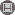Volume 14, Issue 2 (10-2019)                   IJMSI 2019, 14(2): 1-8 | Back to browse issues page

BibTeX | RIS | EndNote | Medlars | ProCite | Reference Manager | RefWorks
Send citation to:Bataineh M, Abu-dawwas R. Graded r-Ideals. IJMSI. 2019; 14 (2) :1-8
URL: http://ijmsi.ir/article-1-984-en.html
Abstract:

Let \$G\$ be a group with identity \$e\$ and \$R\$ be a commutative
\$G\$-graded ring with nonzero unity \$1\$. In this article, we introduce the concept
of graded \$r\$-ideals. A proper graded ideal \$P\$ of a graded ring \$R\$ is said to be
graded \$r\$-ideal if whenever \$a, bin h(R)\$ such that \$abin P\$ and \$Ann(a)={0}\$,
then \$bin P\$. We study and investigate the behavior of graded \$r\$-ideals to introduce
several results. We introduced several characterizations for graded \$r\$-ideals;
we proved that \$P\$ is a graded \$r\$-ideal of \$R\$ if and only if \$aP=aRbigcap P\$
for all \$ain h(R)\$ with \$Ann(a)={0}\$. Also, \$P\$ is a graded \$r\$-ideal of \$R\$
if and only if \$P=(P:a)\$ for all \$ain h(R)\$ with \$Ann(a)={0}\$. Moreover,
\$P\$ is a graded \$r\$-ideal of \$R\$ if and only if whenever \$A, B\$ are graded ideals of
\$R\$ such that \$ABsubseteq P\$ and \$Abigcap r(h(R))neqphi\$, then \$Bsubseteq P\$.
In this article, we introduce the concept of \$huz\$-rings. A graded ring \$R\$
is said to be \$huz\$-ring if every homogeneous element of \$R\$ is either a zero
divisor or a unit. In fact, we proved that \$R\$ is a \$huz\$-ring if and only
if every graded ideal of \$R\$ is a graded \$r\$-ideal. Moreover, assuming that
\$R\$ is a graded domain, we proved that \${0}\$ is the only graded \$r\$-ideal of \$R\$.

Type of Study: Research paper | Subject: Special